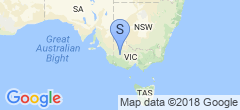## Trigonometry for Pilot Vehicle Signs

Dad rang me up this morning with an interesting problem, and I’m ashamed to say it took me most of the day to get this straight in my head so I could solve it. I think I have the math correct. Basically, you have a sign that’s about 600mm tall, which pivots on the roof rack at point $A$. There’s a gas strut, which pivots on the roof rack at point $B$, and the sign at point $C$, completing a simple triangle. With me so far?

The gas strut (or a linear actuator, which one you use is not really relevant to the mathematics behind it, other than that you probably want one with sufficient travel so the force of wind upon the sign at 100KM/h doesn’t overpower it - but that’s mathematics for another day), which makes up length $a$ (opposite point $A$, on the right slope of the triangle on my diagrams) can range in length from $S{min}$ to $S{max}$. The top of our sign must be (per VicRoads and most other state regulations) no more than 200mm back from the bottom - the sign is 600mm tall, which means the sign must be no more than $30\unicode{xb0}$ back from vertical, or $60\unicode{xb0}$ from the horizontal - that’s for angle $A$. We want $A$ to go to zero when $a$ is as close to $S_{min}$ as we can get, for the gas strut/linac to have maximum mechanical advantage.

So what to set $b$ (the distance between the roof pivot and strut pivot on the sign, or the left slope on my diagrams) and $c$ (the distance between the sign pivot and strut pivot on the roof, or the bottom line of the triangle on my diagrams) to satisfy this? That’s what took me so fuckin’ long. We can use the sine law to determine the ratios of the other two angles/lengths based off the ratio of $A$ to $a$:

$\frac{sinA}{a} = \frac{sinB}{b}$

$cosA = \frac{b^2+c^2-a^2}{2bc}$

We don’t really care about the other angles or lengths, but know that ideally we want $c = b + S_{min}$. Wolfram Alpha finally came to my rescue, because I kept screwing up solving for $b$. I think it works thusly (assuming I’ve made no errors transcribing it back to my blog):

$b=\frac{-\sqrt{(cosA-1)(-2{S{max}}^2+{S{min}}^2cosA+{S{min}}^2)} + S{min}(-cosA)+S_{min}}{2(cosA-1)}$

$c=b+S_{min}$

Being that it’s a quadratic, there are two solutions, I picked the negative root one because it was the only one that didn’t return a negative number on the inputs I put in. But basically, you provide the function with the angle $A$ at full excursion, and the $S{min}$ and $S{max}$ values of your strut, the formulae will tell you the rest. I would link to a solution at Wolfram Alpha, but unless you pay for pro it’ll exceed the computation time and won’t give you an answer.

Dad was running out of daylight so we settled on values found by brute force before I finished solving it correctly, but we ended up rather close (with $A=58\unicode{xb0}$). I think the maths works out though - if you set $A = 60$, $S{max}$ = 565, $S{min} = 335$, I come up with ~317mm for $a$ (bolt to bolt up the sign frame) and ~652mm for $c$ (bolt to bolt along the roof rack).

Feel free to email me if I’ve made a huge mistake, but please use small words. :(fwaggle

Published:

Modified:

Filed under:

Location:

Horsham, VIC, Australia